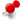# How to create a figure with two graphviz diagrams in it?ClassicListThreaded3 messagesOpen this post in threaded view
|

## How to create a figure with two graphviz diagrams in it?

 Hello, I want to create a figure containing two graphviz diagrams. How can I do this in asciidoc? [graphviz] .... digraph A { } .... [graphviz] .... digraph B { } .... I want the diagrams above to be part of a single figure. Sid
 Graphviz supports two subgraphs like this: ```[graphviz] .... digraph G { compund=true ; subgraph A { a -> b; b -> a; }; subgraph B { rankdir=LR; 1->2; 2->1; }; } .... ``` I hope this will get you closer to your goal. Alexander Schwartz (alexander.schwartz@gmx.net) https://www.ahus1.de